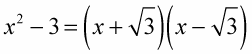##### Linear Algebra For Dummies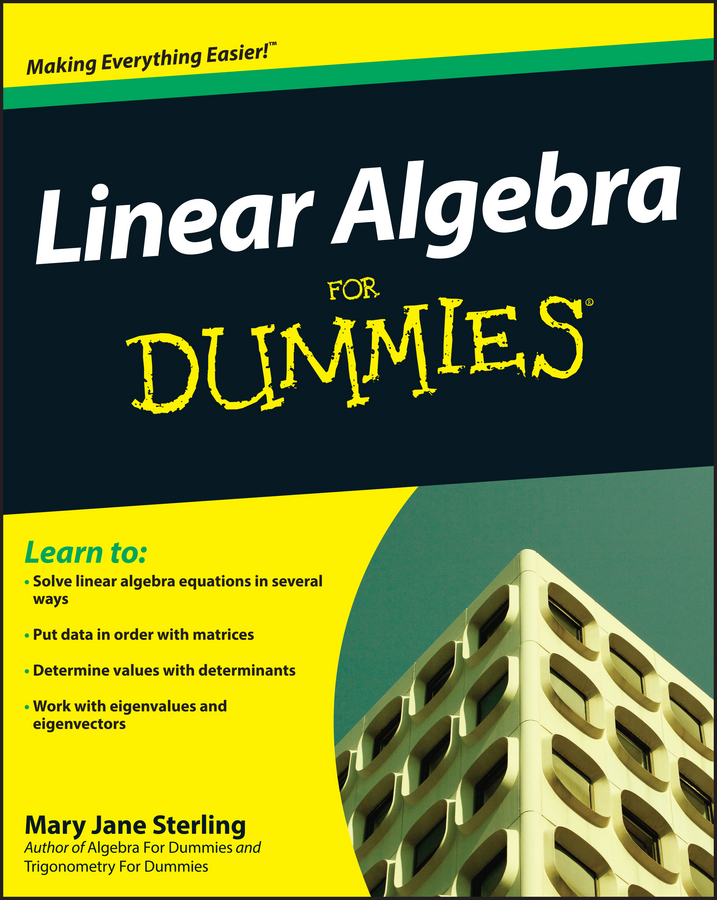If two terms in a binomial are perfect squares separated by subtraction, then you can factor them. To factor the difference of two perfect squares, remember this rule: if subtraction separates two squared terms, then the sum and the difference of the two square roots factor the binomial. For example: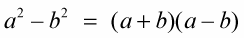Example 1: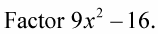1. Find the square roots of the two terms that are perfect squares.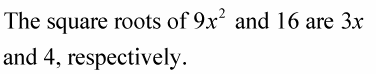2. Write the factorization as the sum and difference of the square roots.

The sum of the roots is 3x + 4 and the difference between the roots is 3x – 4.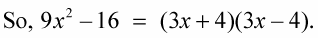Example 2: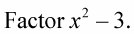1. Find the square roots of the two terms that are perfect squares.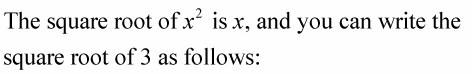Just place the number inside the square root symbol.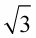2. Write the factorization as the sum and difference of the square roots.